## 20070928

### Physics clicker question: equal magnitude, opposite direction forces

Physics 5A, Fall Semester 2007
Cuesta College, San Luis Obispo, CA

Cf. Giambattista/Richardson/Richardson, Physics, 1/e, Problem 4.50

Students were asked the following clicker questions (Classroom Performance System, einstruction.com) near the beginning of their learning cycle: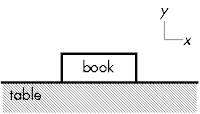[0.6 participation points.] Which Newton's law tells you that the force of the Earth pullling on the book is equal in magnitude and opposite in direction to the force of the table pushing on the book?
(A) Newton's first law.
(B) Newton's second law.
(C) Newton's third law.
(D) (I'm lost, and don't know how to answer this.)

Sections 0906, 0907
(A) : 25 students
(B) : 4 students
(C) : 10 students
(D) : 1 student

The force of the Earth pullling on the book and the force of the table pushing on the book sum to zero, as Newton's first law applies to the book.

After discussion, a related follow-up question was asked, with the following results.

[0.6 participation points.] Which Newton's law tells you that the force of the book pushing on the table is equal in magnitude and opposite in direction to the force of the table pushing on the book?
(A) Newton's first law.
(B) Newton's second law.
(C) Newton's third law.
(D) (I'm lost, and don't know how to answer this.)

Sections 0906, 0907
(A) : 25 students
(B) : 3 students
(C) : 11 students
(D) : 1 student

The force of the book pushing on the table and the force of the table pushing on the book satisfy all three components of the POFOSTITO mnemonic (cf. Light and Matter by Benjamin Crowley, lightandmatter.com):

POF: Pair of Opposite Forces
OST: Of Same Type (in this case, normal forces)
ITO: Involving Two Objects (table and book)

Thus the force of the book pushing on the table and the force of the table pushing on the book are a third law pair, and are equal in magnitude and opposite in direction due to Newton's third law.

At this point in the learning cycle, students were already familiar with Newton's first law, but had trouble engaging their just-learned knowledge of Newton's third law in recognizing third law pairs.

## 20070927

### Physics clicker questions: contact force sequence

Physics 5A, Fall Semester 2007
Cuesta College, San Luis Obispo, CA

Cf. Giambattista/Richardson/Richardson, Physics, 1/e, Problem 4.51

Students were asked the following clicker questions (Classroom Performance System, einstruction.com) near the beginning of their learning cycle:

Mass of book = 0.50 kg.
Coefficient of static friction mu_s = 0.45.
Coefficient of kinetic friction mu_k = 0.40.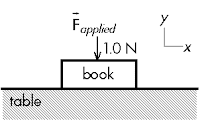[0.6 participation points.] You push down on the book with a force of 1.0 N. The normal force of the table on the book has a magnitude of:
(A) 4.9 N.
(B) 1.0 N.
(C) 4.9 N – 1.0 N.
(D) 4.9 N + 1.0 N.
(E) (I'm lost, and don't know how to answer this.)

Sections 0906, 0907
(A) : 2 students
(B) : 22 students
(C) : 2 students
(D) : 9 students
(E) : 0 students

This question was asked again after displaying the tallied results with the lack of consensus, with the following results. No comments were made by the instructor, in order to see if students were going to be able to discuss and determine the correct answer among themselves.

Sections 0906, 0907
(A) : 1 student
(B) : 18 students
(C) : 2 students
(D) : 15 students
(E) : 0 students

The book has the weight force of the Earth pulling down on it (4.9 N), the applied force (1.0 N) pushing down on it, and since Newton's first law applies, the normal force of the table pushing up on the book is 5.9 N in magnitude.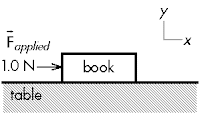[0.6 participation points.] You push to the right on the book with a force of 1.0 N. The static friction force of the table on the book has a magnitude of:
(A) 4.9 N.
(B) 1.0 N.
(C) 2.0 N.
(D) 2.2 N.
(E) (I'm lost, and don't know how to answer this.)

Sections 0906, 0907
(A) : 10 students
(B) : 8 students
(C) : 7 students
(D) : 12 students
(E) : 0 students

The maximum static friction force is mu_s*N = 2.2 N. Since the applied force is less than this, the book remains stuck, Newton's first law applies, and the static friction force is equal in magnitude and opposite in direction to the applied force.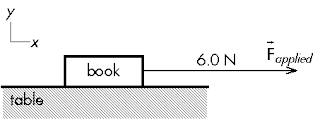[0.6 participation points.] You pull to the right on the book with a force of 6.0 N. The kinetic friction force of the table on the book has a magnitude of:
(A) 4.9 N.
(B) 6.0 N.
(C) 2.0 N.
(D) 2.2 N.
(E) (I'm lost, and don't know how to answer this.)

Sections 0906, 0907
(A) : 1 student
(B) : 4 students
(C) : 28 students
(D) : 4 students
(E) : 0 students

Since the applied force is more than the maximum static friction force magnitude mu_s*N = 2.2 N, the book is effectively unstuck, and begins to slide, thus kinetic friction begins to act on the book, which always has the magnitude mu_k*N = 2.0 N.

## 20070926

### Erasing slate: P-dog shoutout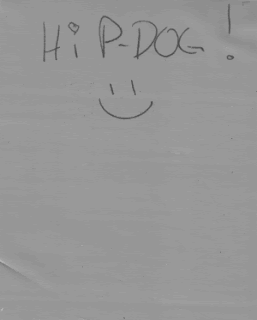"Hi P-DOG! :)" by Anonymous
Fall Semester 2007
Cuesta College, San Luis Obispo, CA

Latest scribbling on the lift-and-erase slate in the hallway, outside the office door.

## 20070925

### Physics clicker question: vector addition of forces

Physics 5A, Fall Semester 2007
Cuesta College, San Luis Obispo, CA

Cf. Giambattista/Richardson/Richardson, Physics, 1/e, Chapter 4.1

Students were asked the following clicker questions (Classroom Performance System, einstruction.com) near the beginning of their learning cycle:

Two forces act on an object, as shown below.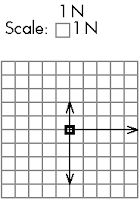[0.6 participation points.] The horizontal component of the net force acting on the object is _____ N.

[0.6 participation points.] The vertical component of the net force acting on the object is _____ N.

Correct answers: F_net,x = 5 N, F_net,y = -2 N.

Student responses (open-ended numerical responses, unpaired)
Sections 0906, 0907
F_net,x = 4 N: 2 students
F_net,x = 5 N: 37 students
F_net,x = 30 N: 1 student

F_net,y = -18 N: 1 student
F_net,y = -3 N: 2 students
F_net,y = -2 N: 35 students
F_net,y = 2 N: 1 student

Two forces act on an object, as shown below.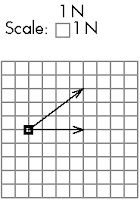[0.6 participation points.] The horizontal component of the net force acting on the object is _____ N.

[0.6 participation points.] The vertical component of the net force acting on the object is _____ N.

Correct answers: F_net,x = 8 N, F_net,y = 3 N.

Student responses (open-ended numerical responses, unpaired)
Sections 0906, 0907
F_net,x = 4 N: 21 students
F_net,x = 6 N: 2 students
F_net,x = 8 N: 15 students

F_net,y = 0.03 N: 1 student
F_net,y = 0.75 N: 1 student
F_net,y = 1.5 N: 1 student
F_net,y = 2 N: 1 student
F_net,y = 3 N: 32 students
F_net,y = 4 N: 1 student
F_net,y = 8 N: 1 student

When orthogonal forces are drawn tail-to-tail, students are relatively successful in summing horizontal and vertical components of the net force. However, the second example demonstrates students having difficulty with vector addition, apparently because they are "completing the triangle" to find the resulting net force.

## 20070924

### Astronomy midterm question: correcting the National Geographic Society

Astronomy 10 Midterm 1, Fall Semester 2007
Cuesta College, San Luis Obispo, CA

Astronomy 10 learning goal Q3.2

[15 points.] Consider the following excerpt (Paul Raeburn, Uncovering the Secrets of the Red Planet: Mars, National Geographic Society, Washington, D.C., 1998, p. 30) regarding Copernicus' heliocentric model of planetary motion:
Copernicus had begun the job of dethroning Ptolemy, but he hadn't quite finished the work. And this is what brings us back to Mars. For all the strengths of the Copernican system, it failed to explain the motion of Mars, including its occasional, peculiar backwards march across the constellations of the zodiac.
Discuss why this statement is wrong. Support your answer using a diagram showing how Copernicus' model does explain the "occasional, peculiar backwards" motion of Mars.

• p = 15/15: Draws a diagram with the Sun at the center (Copernicus' heliocentric model of planetary motion), showing how the Earth (moving faster in an inner orbit) "lapping" Mars (moving slower in an outer orbit) explains the retrograde motion of Mars.
• r = 12/15:
Nearly correct (explanation weak, unclear or only nearly complete); includes extraneous/tangential information; or has minor errors. Understands how the "lapping" illusion explains retrograde motion, but does not explicitly draw a diagram, or explanation/diagram has minor errors/omissions.
• t = 9/15:
Contains right ideas, but discussion is unclear/incomplete or contains major errors. At least demonstrates understanding of Copernicus' heliocentric model with a diagram or explanation.
• v = 6/15:
Limited relevant discussion of supporting evidence of at least some merit, but in an inconsistent or unclear manner. Explains retrograde motion using Ptolemy's geocentric model.
• x = 3/15:
Implementation/application of ideas, but credit given for effort rather than merit. Missing or inapplicable diagram. Problematic explanation of Ptolemy's geocentric model.
• y = 1.5/15:
Irrelevant discussion/effectively blank.
• z = 0/15:
Blank.
Section 1073
p: 19 students
r: 2 students
t: 4 students
v: 19 students
x: 4 students
y: 0 students
z: 0 students

A sample of a "p" response (from J. A.):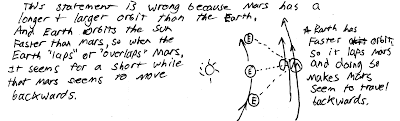## 20070921

### Astronomy midterm question: prograde Moon

Astronomy 10 Midterm 1, Fall Semester 2007
Cuesta College, San Luis Obispo, CA

Astronomy 10 learning goal Q2.1

[15 points.] An observer in San Luis Obispo, CA notices that the Moon goes through prograde (proper) motion each night, with respect to the background stars. Explain why this is so, using a diagram showing the positions and motions of an observer, the Moon, and the Earth.

• p = 15/15: Correct. Draws a diagram showing that the from night-to-night, the stars will rise at approximately the same time (with a few minutes), due to the Earth rotating on its axis. However, from night-to-night, the Moon will move along its ccw orbit around the Earth, and thus appear to be in a slightly different position with respect to the stars. Diagram and/or accompanying explanation is convincing and clear.
• r = 12/15:
Nearly correct (explanation weak, unclear or only nearly complete); includes extraneous/tangential information; or has minor errors. Diagram demonstrates how relative speeds of Earth rotating and Moon orbiting Earth cause the Moon to appear in different parts of the sky from night-to-night, but directions may be reversed, or discussion may have minor omissions.
• t = 9/15:
Contains right ideas, but discussion is unclear/incomplete or contains major errors. Diagram/discussion points out differences between motions of Earth rotation and orbiting Moon, but does not fully demonstrate how the relative speeds of these motions causes night-to-night prograde motion of the Moon, relative to the background stars. May argue that the Moon undergoes prograde motion, because it is never experiences retrograde motion, due to the Moon never being "lapped" by the Earth.
• v = 6/15:
Limited relevant discussion of supporting evidence of at least some merit, but in an inconsistent or unclear manner. Diagram is incomplete, or has wrong or no motion(s) indicated, with limited corresponding discussion.
• x = 3/15:
Implementation/application of ideas, but credit given for effort rather than merit. Missing or inapplicable diagram.
• y = 1.5/15:
Irrelevant discussion/effectively blank.
• z = 0/15:
Blank.
Section 0135
p: 2 students
r: 5 students
t: 19 students
v: 13 students
x: 0 students
y: 1 students
z: 0 students

A sample of a "p" response (from J. G.):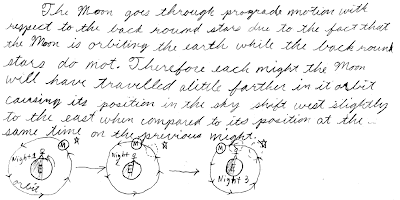## 20070920

### Newton's laws flowcharts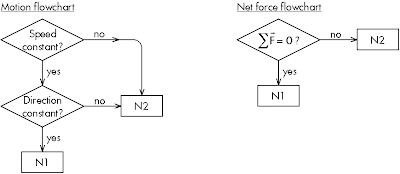"Newton's Laws Flowcharts," Patrick M. Len
September 15, 2007

Flowcharts detailing the decision tree in determining which Newton's laws apply to a particular situation, whether by looking at motion criteria, or at net force criterion. Students later make connections between the equilibrium and non-equilibrium aspects of motion with the zero or non-zero net force cases in formally defining Newton's first and second laws.

A student asked if these flowcharts only apply in outer space (i.e., free from any "real forces"); they apply to any and all situations (that can be observed from an observer in a inertial reference frame).

## 20070919

### Physics quiz question: final speed of falling object

Physics 5A Quiz 2, Fall Semester 2007
Cuesta College, San Luis Obispo, CA

Cf. Giambattista/Richardson/Richardson, Physics, 1/e, Problem 2.43

[3.0 points.] A brick is thrown and released vertically upward with an initial speed of 2.00 m/s from the top ledge of a building, 12.0 m above ground level. The brick reaches its highest height, then moves downwards to strike the ground. Take upwards to be the positive vertical direction. With what speed does the brick strike the ground?
(A) 10.8 m/s.
(B) 11.0 m/s.
(C) 15.3 m/s.
(D) 15.5 m/s.

Since time is not explicitly given, the most direct approach is to solve for v_f,y using:

(v_fy)^2 - (v_iy)^2 = 2*a_y*delta(y),

where v_iy = +2.00 m/s, a_y = -9.80 m/s^2, and delta(y) = -12.0 m.

Response (C) is using v_iy = 0 (this was the most common error). Response (B) is leaving out the factor of 2. Response (A) is the result of both errors in responses (C) and (B).

Student responses
Sections 0906, 0907
(A) : 1 student
(B) : 5 students
(C) : 20 students
(D) : 16 students

## 20070918

### Physics quiz question: freefall velocity versus time graph

Physics 5A Quiz 2, Fall Semester 2007
Cuesta College, San Luis Obispo, CA

Cf. Giambattista/Richardson/Richardson, Physics, 1/e, Problem 2.43

[3.0 points.] A brick is thrown and released vertically upward with an initial speed of 2.00 m/s from the top ledge of a building, 12.0 m above ground level. The brick reaches its highest height, then moves downwards to strike the ground. Take upwards to be the positive vertical direction. Which is the graph of v_y(t) for the brick? Clearly circle your choice below.?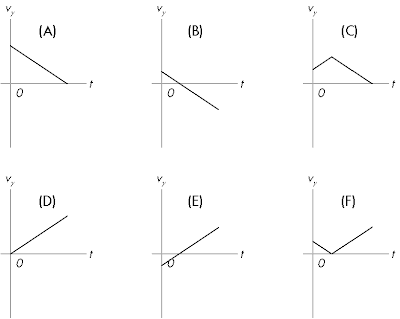With positive +y direction being upwards, the acceleration of an object in free fall is a_y = -9.80 m/s^2, which must be the slope of the v_y(t) graph. Graph (E) is correct except that the positive +y direction is downwards. Graph (C), the most common error, resembles in a crude manner the parabolic y(t) graph of an object ascending, and then descending down below its initial position above the ground.

Student responses
Sections 0906, 0907
(A) : 1 student
(B) : 5 students
(C) : 23 students
(D) : 1 student
(E) : 9 students
(F) : 12 students

## 20070917

### Physics quiz question: change in velocity

Physics 5A Quiz 2, Fall Semester 2007
Cuesta College, San Luis Obispo, CA

Cf. Giambattista/Richardson/Richardson, Physics, 1/e, Conceptual Question 2.5

[3.0 points.] The change in (instantaneous) velocity is:
(A) the area under a v_x(t) graph.
(B) the area under an a_x(t) graph.
(C) the tangent slope of an x(t) graph.
(D) the tangent slope of a v_x(t) graph.

(Cf. "Physics chain of pain," an earlier post summarizing the graphical slope-area relations between kinematics graphs.)

Response (A) is the displacement delta(x); response (C) is the (instantaneous) velocity; response (D) is the (instantaneous) acceleration.

Student responses
Sections 0906, 0907
(A) : 2 students
(B) : 11 students
(C) : 16 students
(D) : 13 students

## 20070914

### Astronomy quiz question: Foucault pendulum

Astronomy 10 Quiz 3, Fall Semester 2007
Cuesta College, San Luis Obispo, CA

Astronomy 10 learning goal Q3.4

[3.0 points.] Which one of the following choices best explains what the Foucault pendulum demonstrates in order to disprove geocentrism and/or prove heliocentrism?
(A) The Earth spins on an axis.
(B) Kepler's laws must be true.
(C) Circular epicycles cannot accurately predict positions of the planets.
(D) The Earth has an oblate (squashed) shape.
(E) The Earth is orbiting the Sun.

The direction of a Foucault pendulum's swing will remain stationary while the Earth rotates with respect to it, providing evidence that the Earth rotates on its own axis.

Student responses
Section 0135
(A) : 11 students
(B) : 4 students
(C) : 7 students
(D) : 1 student
(E) : 14 students

## 20070913

### Astronomy quiz question: aberration of starlight

Astronomy 10 Quiz 3, Fall Semester 2007
Cuesta College, San Luis Obispo, CA

Astronomy 10 learning goal Q3.4

[3.0 points.] Which one of the following choices best explains what the aberration of starlight demonstrates in order to disprove geocentrism and/or prove heliocentrism?
(A) The Earth spins on an axis.
(B) Kepler's laws must be true.
(C) Circular epicycles cannot accurately predict positions of the planets.
(D) The Earth has an oblate (squashed) shape.
(E) The Earth is orbiting the Sun.

The direction of the aberration of starlight varies over the course of a year, which provides evidence that the Earth is not stationary, but is orbiting the Sun.

Student responses
Section 1073
(A) : 11 students
(B) : 7 students
(C) : 15 students
(D) : 1 student
(E) : 16 students

## 20070912

### Astronomy current events question: Spirit and Opportunity rover status

Astronomy 10L, Fall Semester 2007
Cuesta College, San Luis Obispo, CA

Students are assigned to read online articles on current astronomy events (skytonight.com, from Sky & Telescope magazine), and take a short current events quiz during the first 10 minutes of lab. (This motivates students to show up promptly to lab, as the time cut-off for the quiz is strictly enforced!)

[0.2 points.] What is the current condition of the NASA Mars Expedition Rovers Spirit and Opportunity? Circle your answer below.
(A) They are tilting their solar panels back and forth to slide off dust storm debris.
(B) They are rolling their wheels back and forth in order to unstick themselves from being half-buried in dust storm debris.
(C) They can now collect enough solar energy to move around and communicate daily with Earth.
(D) Currently out of direct radio contact for the next two months, as Mars is on the far side of the Sun.
(E) Unknown, as all transmissions ended abruptly last month during a planet-wide dust storm.

Student responses
Section 0139
(A) : 4 students
(B) : 9 students
(C) : 15 students
(D) : 3 student
(E) : 1 students

## 20070911

### Lolcat: ignoring gravity"I'm running down ur wallz..." by clive
icanhascheezburger.com
June 25, 2007

Physics 8A learning goal Q3.4

Running down a wall does not necessarily mean gravity has been ignored...but nice try!

## 20070910

### Astronomy quiz question: full Moon rising/setting

Astronomy 10 Quiz 2, Fall Semester 2007
Cuesta College, San Luis Obispo, CA

Astronomy 10 learning goal Q2.2

[Version 1]

[3.0 points.] Consider the view of the western horizon shown below, as seen from San Luis Obispo, CA at a certain time of day (or night). Carefully note the position and phase of the Moon in its full phase.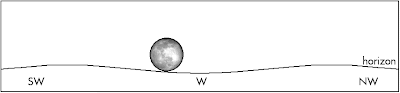Which one of the following choices best describes time of day (or night) that an observer in San Luis Obispo, CA will see the view shown above?
(A) Just before sunrise.
(B) Just after sunrise.
(C) Just before sunset.
(D) Just after sunset.
(E) (It is not possible to determine the time of day for the view shown above, unless more information is given.)

The full Moon sets in the west just as the sun rises (in the east). Since the Moon is just above the western horizon, before setting, then this must be just before the sun rises from the east horizon.

Student responses
Section 0135
(A) : 7 students
(B) : 1 student
(C) : 7 students
(D) : 4 students
(E) : 0 students

Section 1073
(A) : 7 students
(B) : 5 students
(C) : 8 students
(D) : 3 students
(E) : 4 students

[Version 2]

[3.0 points.] Consider the view of the western horizon shown below, as seen from San Luis Obispo, CA at a certain time of day (or night). Carefully note the position and phase of the Moon in its full phase.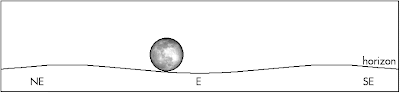Which one of the following choices best describes time of day (or night) that an observer in San Luis Obispo, CA will see the view shown above?
(A) Just before sunrise.
(B) Just after sunrise.
(C) Just before sunset.
(D) Just after sunset.
(E) (It is not possible to determine the time of day for the view shown above, unless more information is given.)

The full Moon rises in the east just as the sun sets (in the west). Since the Moon is just above the eastern horizon, after rising, then this must be just after the sun has set in the west horizon.

Student responses
Section 0135
(A) : 3 students
(B) : 3 students
(C) : 2 students
(D) : 11 students
(E) : 1 student

Section 1073
(A) : 3 students
(B) : 4 students
(C) : 5 students
(D) : 10 students
(E) : 0 students

Interesting to note that students had a lower success rate in answering the version with the sun about to set in the west, compared to the version with the sun just after rising in the east.

## 20070907

### FCI pre-test comparison: Cuesta College versus UC-Davis

Students at both Cuesta College (San Luis Obispo, CA) and the University of California at Davis were administered the Force Concept Inventory (Doug Hestenes, et al.) during the first week of instruction.
`     Cuesta College    UC-Davis     Physics 5A        Physics 7B     Fall Semester     Summer Session II     2007              2002N    35 students       76 studentslow   3                 2mean  9.1 +/- 3.9       9.1 +/- 4.1high 19                27`

A "Student" t-test of the null hypothesis results in p = 0.97, thus there is no significant difference between Cuesta College and UC-Davis FCI pre-test scores.

The Physics 5A students at Cuesta College found these preliminary results interesting--statistically speaking, they are as knowledgeable about the Newtonian physics concepts covered in the FCI as UC-Davis students at the start of introductory college physics.

Later this semester (Fall 2007), a comparison will be made between Cuesta College and UC-Davis FCI post-tests, along with their pre- to post-test gains.

D. Hestenes, M. Wells, and G. Swackhamer, Arizona State University, "Force Concept Inventory," Phys. Teach. 30, 141-158 (1992).
Development of the FCI, a 30-question survey of basic Newtonian mechanics concepts.

## 20070906

### Astronomy quiz question: non-circumpolar and circumpolar constellations

Astronomy 10 Quiz 1, Fall Semester 2007
Cuesta College, San Luis Obispo, CA

Astronomy 10 learning goal Q1.3

[Version 1]

[3.0 points.] According to your starwheel, which one of the following choices is not a circumpolar constellation, as seen by an observer in San Luis Obsipo, CA?
(A) Camelopardalis.
(B) Cassiopeia.
(C) Cepheus.
(D) Lacerta.
(E) Ursa Minor.

Moving the dial on a starwheel (planisphere) shows that while the other constellations stay above the horizon, Lacerta dips below the horizon, thus making it a non-circumpolar constellation.

Student responses
Section 1073
(A) : 0 students
(B) : 0 students
(C) : 3 students
(D) : 42 students
(E) : 9 students

[Version 2]

[3.0 points.] According to your starwheel, which one of the following choices is a circumpolar constellation, as seen by an observer in San Luis Obsipo, CA?
(A) Auriga.
(B) Boötes.
(C) Camelopardalis.
(D) Gemini.
(E) Lacerta.

Moving the dial on a starwheel (planisphere) shows that while the other constellations eventually dip below the horizon, Camelopardalis always stays above the horizon, thus making it a circumpolar constellation.

Student responses
Section 0135
(A) : 1 student
(B) : 3 students
(C) : 30 students
(D) : 8 students
(E) : 0 students

Apparently students have the same rate of success in distinguishing non-circumpolar constellations from circumpolar constellations, and vice versa.

## 20070904

### Physics quiz question: density

Physics 5A Quiz 1, Fall Semester 2007
Cuesta College, San Luis Obispo, CA

Cf. Giambattista/Richardson/Richardson, Physics, 1/e, Problem 1.14

[3.0 points.] Density is the ratio of mass to volume. Ocean water has a density of 1.027 kg/m^3. What is the density of ocean water in units of g/cm^3?
(A) 1.027 x 10^(-3) g/cm^3.
(B) 1.027 g/cm^3.
(C) 10.27 g/cm^3.
(D) 1.027 x 10^3 g/cm^3.

1 kg = 1,000 g; 1 m = 100 cm.

Then 1.027 kg/m^3
= (1.027 kg/m^3)*(1,000 g/1 kg)*(1 m/100 cm)*(1 m/100 cm)*(1 m/100 cm)
=1.027 x 10^(-3) g/cm^3.

The incorrect response (C) has (1,000 g/1 kg), but only one conversion factor of (1 m/100 cm).

Student responses
Sections 0906, 0907
(A) : 18 students
(B) : 5 students
(C) : 16 students
(D) : 4 students

## 20070903

### Physics quiz question: radial acceleration

Physics 5A Quiz 1, Fall Semester 2007
Cuesta College, San Luis Obispo, CA

Cf. Giambattista/Richardson/Richardson, Physics, 1/e, Comprehensive Problem 1.51

[3.0 points.] An object of mass m moving at constant speed v around a circle of radius r has a radial acceleration a = mv^2/r. If the speed is increased 12.0%, by what percentage does the radial acceleration increase?
(A) 1.44%.
(B) 13.6%.
(C) 25.4%.
(D) 29.1%.

a_1 = m*(v_1)^2/r, and a_2 = m*(v_2)^2/r. With v_2 = 1.120*v_1, then:

a_2/a_1 = (m*(v_2)^2/r)/(m*(v_1)^2/r) = (v_2/v_1)^2 = (1.120*v_1/v_1)^2 = (1.120)^2 = 1.2544,

or an increase of 25.4%, to three significant figures (expressed in percent form).

The incorrect response (A) is 100*(0.12)^2.

Student responses
Sections 0906, 0907
(A) : 16 students
(B) : 4 students
(C) : 23 students
(D) : 0 students